Interview First Aid
Starter's Guide to Case Prep
Crack the Case Interview
Ace the Personal Fit Interview
Master the Problem Solving Test
Case Cracking

# The net present value (NPV) allows you to evaluate future cash flows based on present value of money

The net present value (NPV) is the sum of present values of money in different future points in time. The present value (PV) determines how much future money is worth today. Based on the net present valuation, we can compare a set of projects/ investments with different cash-flows over time. This enables us to quantitatively assess a business' attractiveness using a benchmarking of NPVs.

## The closer future cash flows are to the present the more valuable your money is

The concept is also known as time value of money and we provide two explanations below:

• An intuitive explanation: People will prefer money at present because of risk aversion. Would you rather have \$100 today or in a year? Obviously today, because there is a risk that you may not get that \$100 in a year. In addition, once you have the money, you again have a decision to either spend it right away or wait with spending it.
• A financial explanation: Imagine you have \$100. How much is it worth in a year? If you do not leave the money in your pocket, you usually have the option to put the money in your bank account at a low and almost negligible risk. You will earn interest but may lose value due to inflation. However, the inflation adjusted interest rate may be 2%, in absolute terms \$2. In total your \$100 is worth \$102 after one year. Now, you can calculate backwards: If you have a future value of \$102 in a year, how much is it worth today? It is \$102 divided by 1.02 which results in \$100 again.

## How to calculate net present values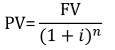• PV is the present value
• FV is the future value
• i is the decimal value of the interest rate for a specific period
• n is the number of periods between present and future

The following is the calculation of the above PV example with \$102 future value at an interest rate of 2%,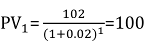Below you can find a slightly different version of the above example, in which you receive \$102 in two years instead of next year. The two year investment earns you a theoretical interest two times, which is why you discount twice.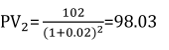The Net Present Value of those two \$102 payments in one and two years is simply its sum.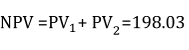## Apply NPV shortcuts to succeed in case situations

It’s unlikely that you will need to calculate a complex NPV during a case interview because the calculations tend to get overly complicated. But in some cases you can apply some shortcuts as discussed below:

### 1) Perpetuity: the NPV for infinite cash flows (meaning business will generate profits for an infinite period of time)

For infinite cash flows, there is a simplified formula: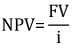Imagine you have to value a company in a case interview. A common approach is to define the value of a company as the sum of all its discounted future profits. If you assume that a company will have the same profits every year for an indefinite time horizon, you just divide the future value of all profits by the respective discount rate. For instance, if you expect the company to yield \$100 every year, the company is worth \$2500 (at a discount rate of 4%).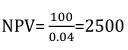To make this more pragmatic, you could assume that the company's profits will grow every year at a certain rate g.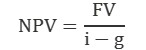Especially for short-term horizons, defining expected growth is difficult. An approximated growth rate for profits that are far into the future is often around 2%. After a while, every business or product life cycle ends up in a competitive market environment and simply grows at the same rate as the overall economy. The above example recalculated with a continuous growth rate of 2% results in a net present value of \$5000 for the company.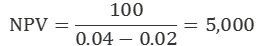Notice that the value is twice the value compared to the calculations without a growth. NPV calculations are very sensitive towards changes of inputs. Therefore, a sensitivity analysis is conducted in most cases. To do so you need to create a range of possible NPVs by using a range of possible growth and discount rates.

### 2. Find the right interest rate i

Finding the correct discounting factor for NPV calculations is the business of entire banking departments. In general, there is one basic rule: the bigger the risk the higher the discount rate.

The rationale behind this rule is simple: The less you can be sure about receiving future earnings, the less you value them. By increasing the discount rate, the NPV of future earnings will shrink. Discount rates for quite secure cash-streams vary between 1% and 3%, but for most companies, you use a discount rate between 4% - 10% and for a speculative start-up investment, the applied interest rate could reach up to 40%. In case interviews, you could ask for the discount rate directly or estimate it at 10% for most scenarios if the interviewer requests you to approximate it.

## Key takeaways

• Use NPVs to evaluate future cash-flows in today’s time value of money.
• By calculating risk-adjusted NPVs, you can quantitatively compare different investments.
• NPVs are used to value a company based on its future profits.

Solve the Gravestone case, an interviewer led case which includes a company valuation

## Related Cases

### Smart Meters

Topics:
Rating:
The municipal utility Hamburg Energized is a local energy retail (power and gas) and distribution grid company active in the city of Hamburg. The majority of shares of Hamburg Energized is held by the city itself. As the distribution system operator of Hamburg, Hamburg Energized is responsible for t ... (Open whole case)
29.8k
Times solved
Intermediate
Difficulty
Rating

### PE Portfolio Strategy

Topics:
Rating:
We are a private equity firm operating primarily in the automotive industry. We would like you to figure out whether we should increase our portfolio in the sensor market or not. We would like you to do a profit/margin growth potential analysis and tell us how we can add value to this com ... (Open whole case)
5.9k
Times solved
Intermediate
Difficulty
Rating
Interview First Aid
Starter's Guide to Case Prep
Crack the Case Interview
Ace the Personal Fit Interview
Master the Problem Solving Test
Case Cracking